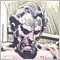Need help with changing ATR to show double its value20

I appreciate help with changing the code of the ATR indicator to show double its value. For example if ATR is 30 pips, I want it to show 60. Thanks!

Here is the code:

//+------------------------------------------------------------------+

//|                                                          ATR.mq4 |

//|                   Copyright 2005-2014, MetaQuotes Software Corp. |

//|                                              http://www.mql4.com |

//+------------------------------------------------------------------+

#property copyright   "2005-2014, MetaQuotes Software Corp."

#property description "Average True Range"

#property strict

//--- indicator settings

#property indicator_separate_window

#property indicator_buffers 1

#property indicator_color1  DodgerBlue

//--- input parameter

input int InpAtrPeriod=20; // ATR Period

//--- buffers

double ExtATRBuffer[];

double ExtTRBuffer[];

//+------------------------------------------------------------------+

//| Custom indicator initialization function                         |

//+------------------------------------------------------------------+

int OnInit(void)

{

string short_name;

//--- 1 additional buffer used for counting.

IndicatorBuffers(2);

IndicatorDigits(Digits);

//--- indicator line

SetIndexStyle(0,DRAW_LINE);

SetIndexBuffer(0,ExtATRBuffer);

SetIndexBuffer(1,ExtTRBuffer);

//--- name for DataWindow and indicator subwindow label

short_name="2xATR("+IntegerToString(InpAtrPeriod)+")";

IndicatorShortName(short_name);

SetIndexLabel(0,short_name);

//--- check for input parameter

if(InpAtrPeriod<=0)

{

Print("Wrong input parameter ATR Period=",InpAtrPeriod);

return(INIT_FAILED);

}

//---

SetIndexDrawBegin(0,InpAtrPeriod);

//---

return(INIT_SUCCEEDED);

}

//+------------------------------------------------------------------+

//| Average True Range                                               |

//+------------------------------------------------------------------+

int OnCalculate(const int rates_total,

const int prev_calculated,

const datetime &time[],

const double &open[],

const double &high[],

const double &low[],

const double &close[],

const long &tick_volume[],

const long &volume[],

{

int i,limit;

//--- check for bars count and input parameter

if(rates_total<=InpAtrPeriod || InpAtrPeriod<=0)

return(0);

//--- counting from 0 to rates_total

ArraySetAsSeries(ExtATRBuffer,false);

ArraySetAsSeries(ExtTRBuffer,false);

ArraySetAsSeries(open,false);

ArraySetAsSeries(high,false);

ArraySetAsSeries(low,false);

ArraySetAsSeries(close,false);

//--- preliminary calculations

if(prev_calculated==0)

{

ExtTRBuffer=0.0;

ExtATRBuffer=0.0;

//--- filling out the array of True Range values for each period

for(i=1; i<rates_total; i++)

ExtTRBuffer[i]=MathMax(high[i],close[i-1])-MathMin(low[i],close[i-1]);

//--- first AtrPeriod values of the indicator are not calculated

double firstValue=0.0;

for(i=1; i<=InpAtrPeriod; i++)

{

ExtATRBuffer[i]=0.0;

firstValue+=ExtTRBuffer[i];

}

//--- calculating the first value of the indicator

firstValue/=InpAtrPeriod;

ExtATRBuffer[InpAtrPeriod]=firstValue;

limit=InpAtrPeriod+1;

}

else

limit=prev_calculated-1;

//--- the main loop of calculations

for(i=limit; i<rates_total; i++)

{

ExtTRBuffer[i]=MathMax(high[i],close[i-1])-MathMin(low[i],close[i-1]);

ExtATRBuffer[i]=ExtATRBuffer[i-1]+(ExtTRBuffer[i]-ExtTRBuffer[i-InpAtrPeriod])/InpAtrPeriod;

}

//--- return value of prev_calculated for next call

return(rates_total);

}

//+------------------------------------------------------------------+3606

m-c-f:

I appreciate help with changing the code of the ATR indicator to show double its value. For example if ATR is 30 pips, I want it to show 60. Thanks!

for(i=limit; i<rates_total; i++)
{
ExtTRBuffer[i]=MathMax(high[i],close[i-1])-MathMin(low[i],close[i-1]);
ExtATRBuffer[i]=ExtATRBuffer[i-1]+(ExtTRBuffer[i]-ExtTRBuffer[i-InpAtrPeriod])/InpAtrPeriod;
ExtATRBuffer[i] *= 2;
}20

Thanks for the reply.

There were no compile errors, but the indicator is not showing any values. The sub window, and the data window are blank. Any ideas? Thanks3606

m-c-f:

the indicator is not showing any values. The sub window, and the data window are blank.

My mistake. This should do the trick:

ExtTRBuffer[i]=MathMax(high[i],close[i-1])-MathMin(low[i],close[i-1]);
ExtTRBuffer[i] *= 2.;
ExtATRBuffer[i]=ExtATRBuffer[i-1]+(ExtTRBuffer[i]-ExtTRBuffer[i-ExtPeriodATR])/ExtPeriodATR;20

That did it. Thanks so much!  Do you know how to get the new indicator to limit the results to 4 decimal places like the original? It is showing 5. Thanks again.3606

m-c-f:
That did it. Thanks so much!  Do you know how to get the new indicator to limit the results to 4 decimal places like the original? It is showing 5. Thanks again.

Do you mean something like this?

IndicatorSetInteger(INDICATOR_DIGITS,4);20

Perfect. It  works exactly as I wanted. Thanks for all your help.